# OECD International Student Mobility Data Analysis 5 - Making a slope chart using R plot(), lines(), points() and text() functions.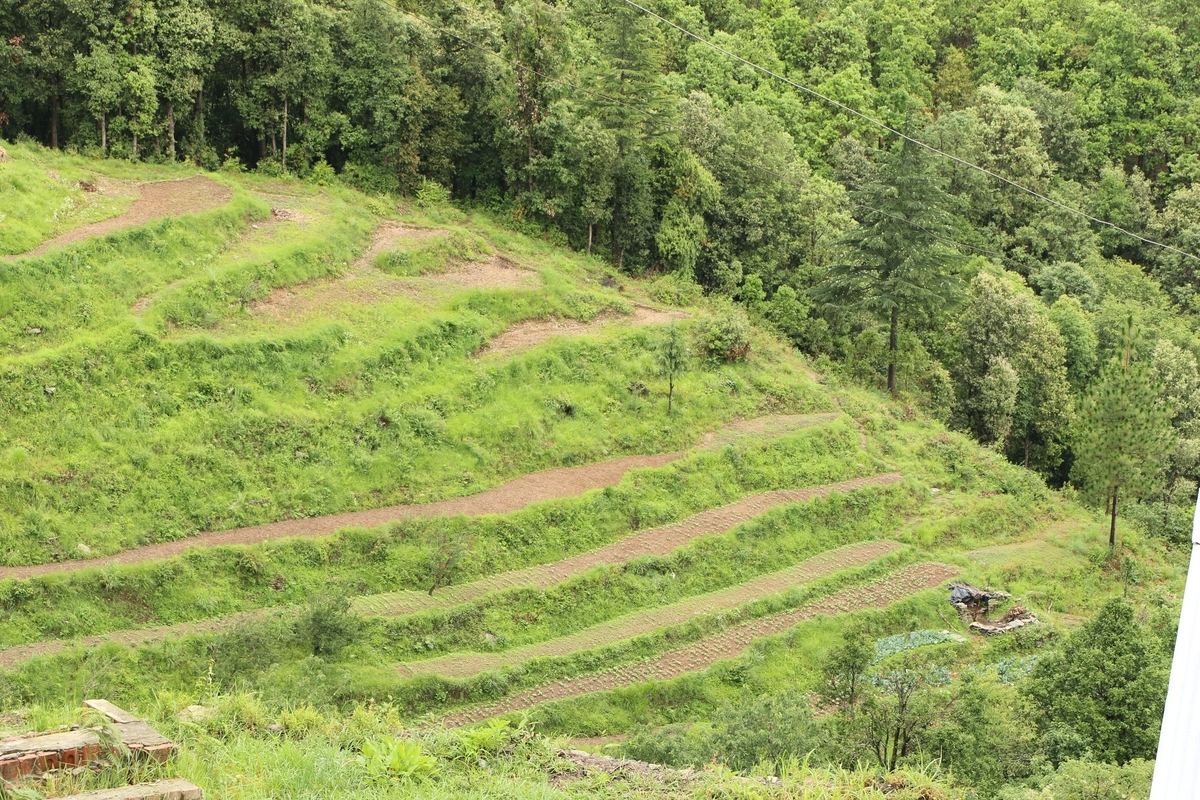Photo by Aditi Jain on Unsplash

This post is following of above post.
In this post, I will make a slope chart using R.
We can get an idea of changes based on the slope of the lines, so we can see which country has improved student mobility very easily.

Let's get started.

First of all, make an empty plot canvas with plot() function.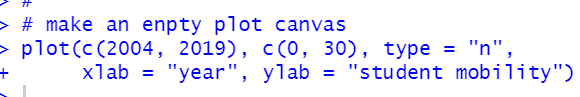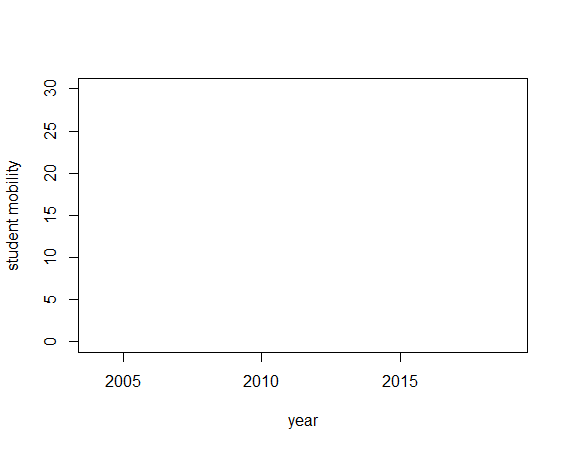Then, draw a start value with points() function.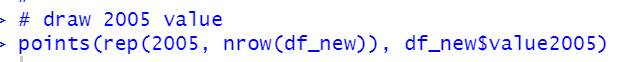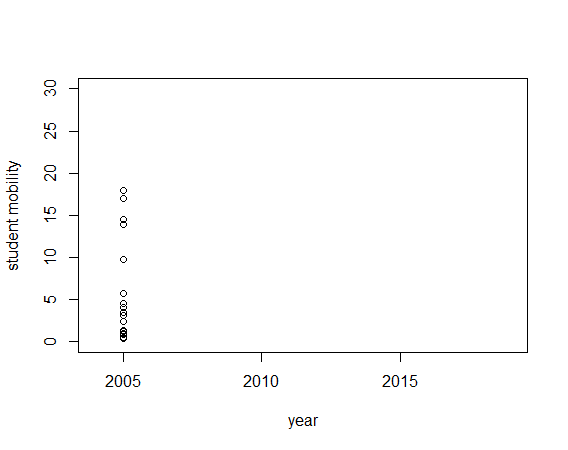Then, add end value with points() function.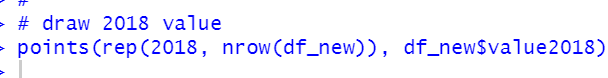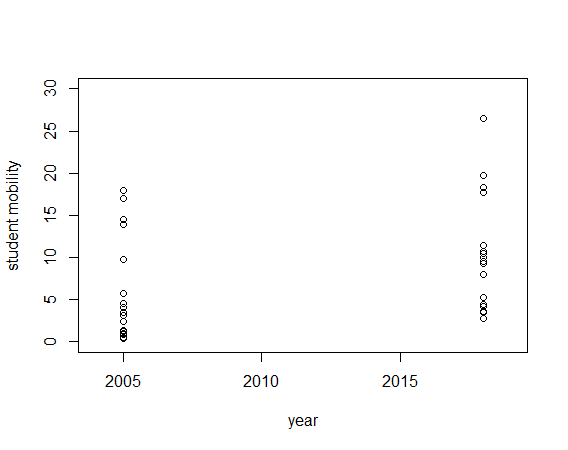Next, using for() and lines() function, we will add slopes.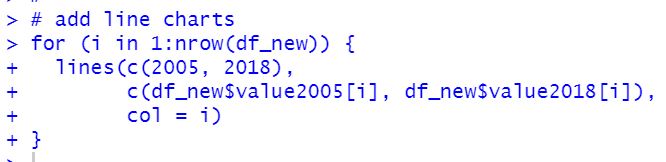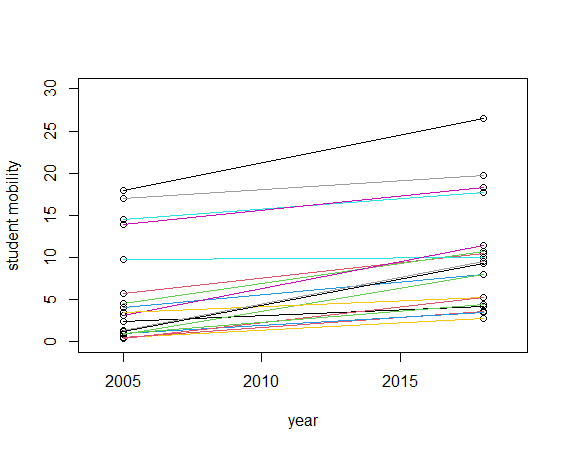Then, add iso code at the left side with text() function.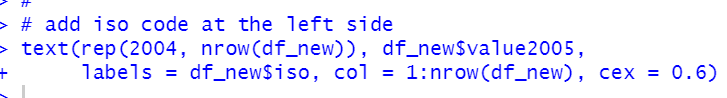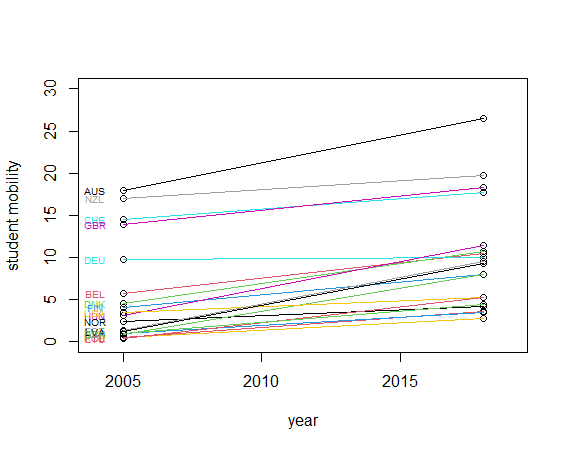Lastly, we will add iso code at the right side too.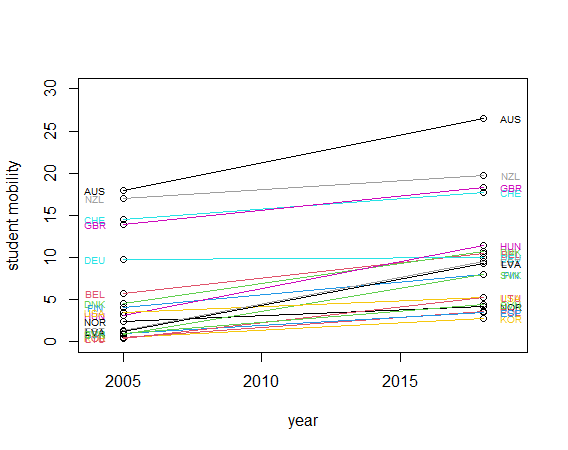All right, We made a slope chart. We will see AUS has largely improved.

That's it. Thank you!